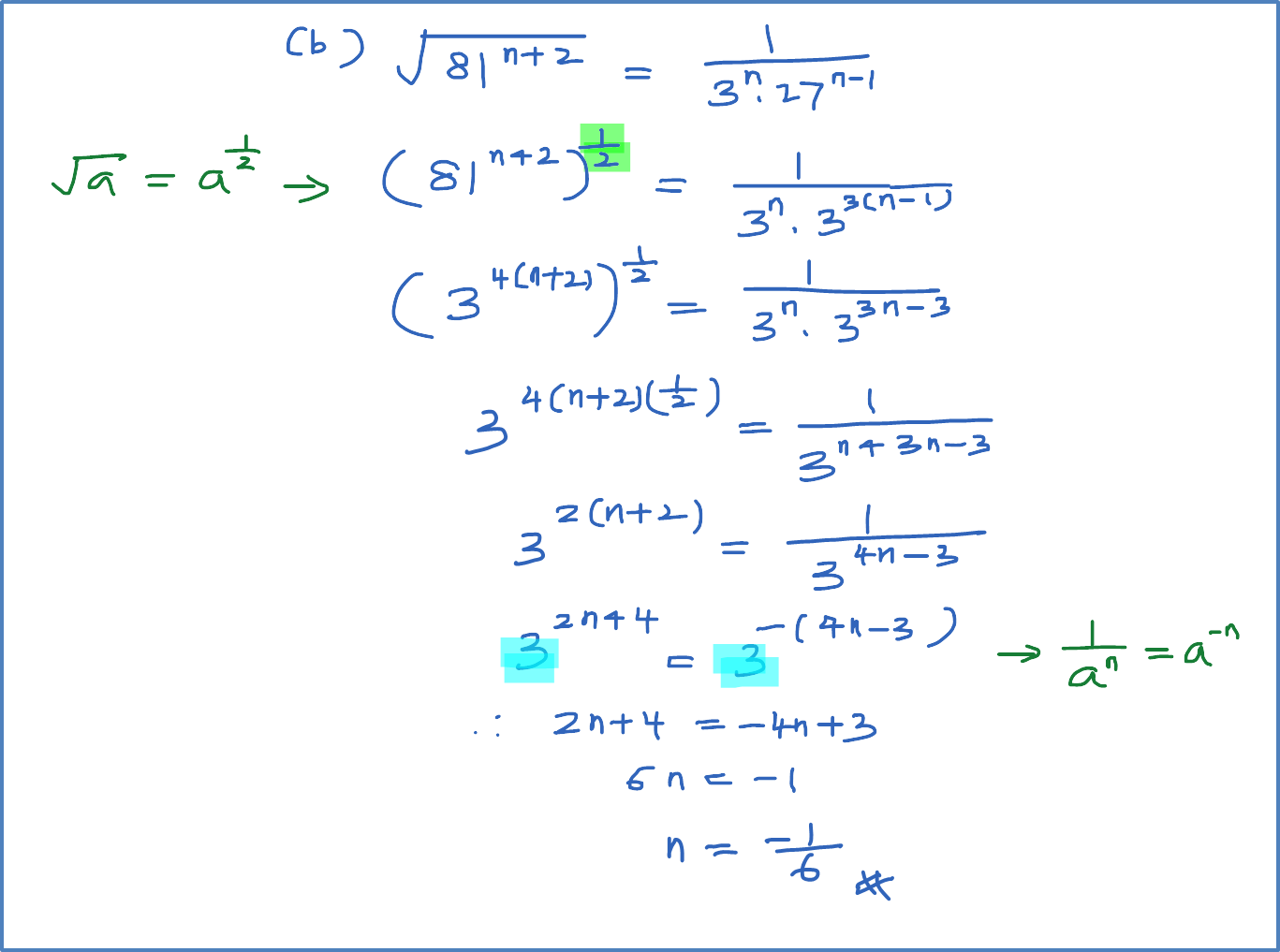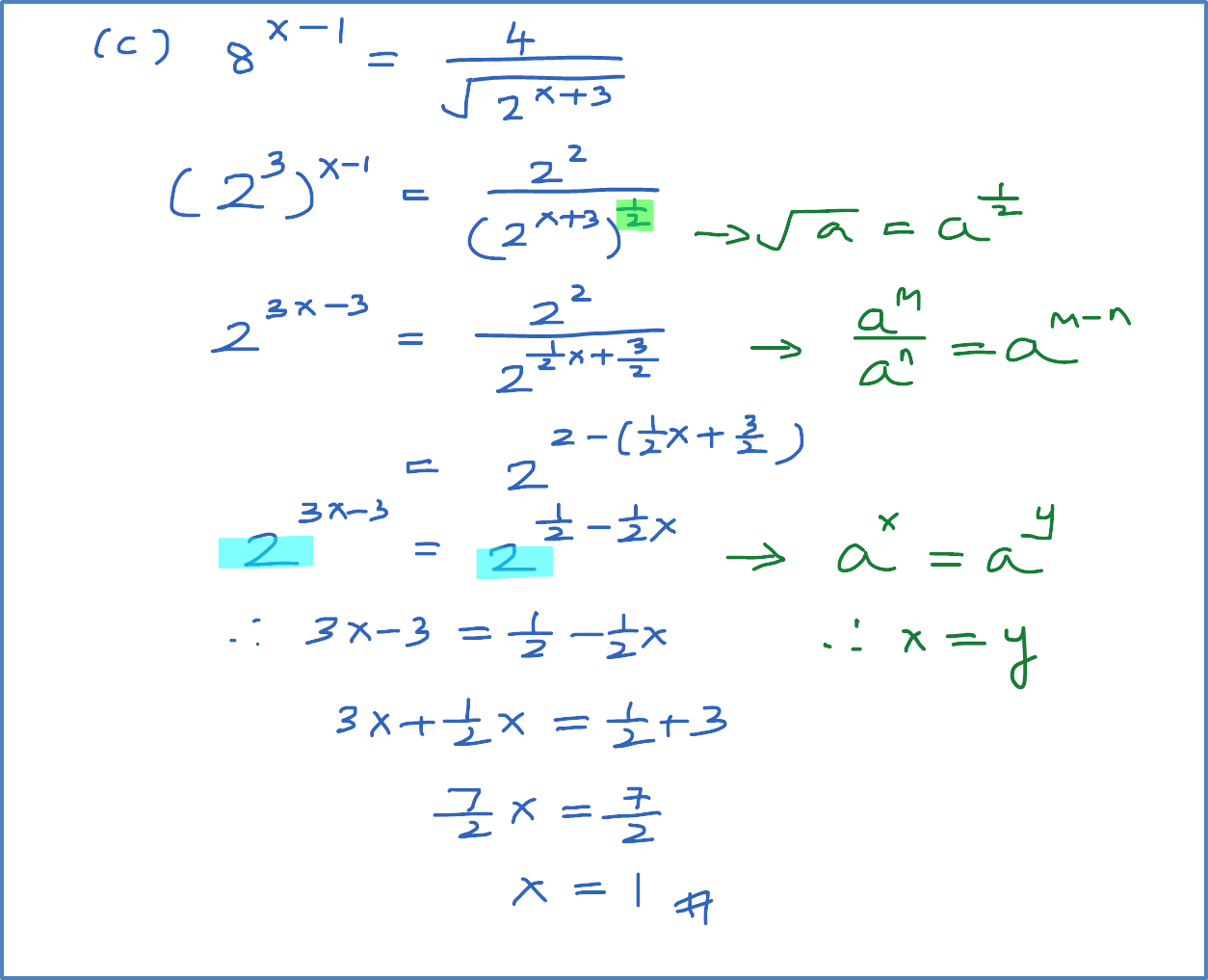4.3.2 Example 2 (Index Equation – Equal Base)

Example 2
Solve each of the following.
(a) $27\left({81}^{3x}\right)=1$
(b) $\sqrt{{81}^{n+2}}=\frac{1}{{3}^{n}{27}^{n-1}}$
(c) ${8}^{x-1}=\frac{4}{\sqrt{{2}^{x+3}}}$